Series

The typical method of finding a series expansion is to enter the complete  expression as in  Series[(x+2)Sin[x],{x,0,5}]. or.  A seldom documented trick is to get the same series expansions using  the cells below.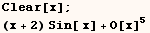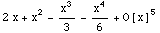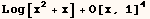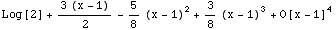You can often use Series to find an asymptotic expansion provided one exists
for the function in question.  An asymptotic expansion is one where the error
gets smaller as the argument approaches infinity.  Series will try to find an
asthmatic expansion if you ask for a series about infinity.  The next cell
gives an asthmatic expansion of Erfc[x].The next cell gives an asthmatic expansion of ArcTan[x].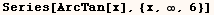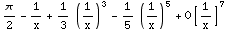It turns out you must first use TrigToExp  to get an asymptotic expansion of
ArcSinnh[x].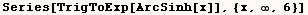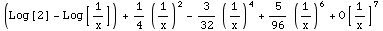You may need to make a power series in two or three variables and truncate  it such that the sum of all exponents in each term is less than or equal to a  certain integer.  If that is the case download a package I have posted at  http://library.wolfram.com/infocenter/MathSource/4950/

Created by Mathematica  (May 16, 2004)

Back to Ted’s Tricks index page